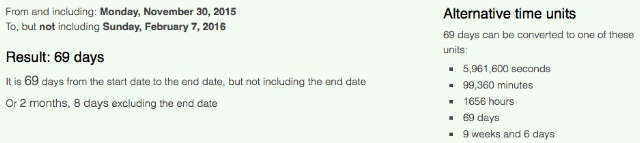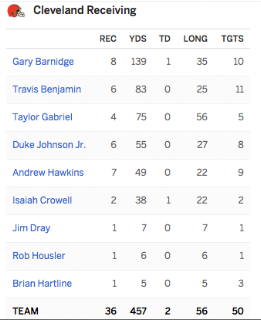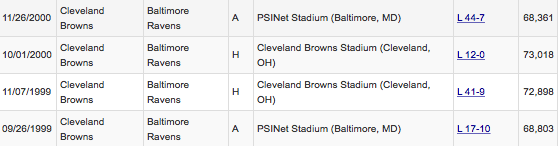## Monday, November 30, 2015

### 77 | Stephen Curry Breaks His Own NBA Record, Making 77 Three Pointers In November, 2015

Notice that Curry made 77 three pointers in the month of November.  There is no doubt this number was scripted.  This is the same amount of three pointers the media reported he made consecutively in practice last April.  Also, keep in mind, the interim coach, Luke Walton, his father won the NBA Finals in the year '77, while playing in the 33rd State.

Also, in the same game wear he set the record for three pointers in a month, his team went on to win with '106' points, the number of prophecy.  The Warriors now have 23 consecutive wins, dating back to April 9, 2015, last season.  Their streak began against the Blazers, that same team from the 33rd State.  Their 33rd win in a row, should it come, will be Christmas Day against LeBron.

### 33 47 51 123 147 | The End of Ravens @ Browns, MNF, November, November 30, 2015

Notice the 42-yard TD reception.  Ohio St. scored 42 points against Jim Harbaugh this weekend, here the Ohio Browns put a 42-yard TD on John Harbaugh, late in the game, with a 1:47 left on the clock. Let us not forget the Harbaugh brothers met in Super Bowl 47, and John prevailed, in the rigged spectacle that was.

Ohio = 15+8+9+15 = 47
John = 10+15+8+14 = 47
John defeated Jim (Harbaugh brothers) in Super Bowl 47

***Super Bowl 47, was the one with the 33-minute blackout

Notice this game tying touchdown came with 1:47 left on the clock.

As for '42', it is an important number as well.  Ohio State won the national championship with that amount of points earlier this year as well.

Freemason = 42 (Pythagorean Gematria)After scoring, to tie the game, the Browns were able to quickly stop the Ravens offense, and got the ball back with 1:23 left in the game (John Harbaugh's 123rd Game as Raven's Coach).  They then drove to the Ravens 33-yard line, emphasis on 33, before attempting a game winning field goal.  Notice how 1:23 is a lot like 123.  In the two games between the two teams this year, the scores were 33-30 and 33-27.  That is a total of 123 points.

Conspiracy = 3+15+14+19+16+9+18+1+3+25 = 123
Conspiracy = 3+6+5+1+7+9+9+1+3+7 = 51 (The final field goals was a 51-yard attempt)

Before the Game, ESPN also let us know about another '123' going on.Joe Flacco = 34; Matt Schaub, 34-years old; 34th game between Browns and Ravens
What is strange about how they ended up on the 33-yard line, is that it seemed the Browns QB purposefully went down early, when he could have gotten another yard or two easily based on where the defensive players were on the field.  In other words, it seems he definitely wanted to go down at the 33.

Remember, this game is on MNF.

MNF = 13+14+6 = 33
Cleveland = 3+3+5+4+5+3+1+5+4 = 33

The last time the teams played, it was their 33rd matchup, and the Browns won 33-30.

ESPN gives us the yardage situation, just before the already infamous in Cleveland, field goal attempt.

Next, the field goal attempt from the 33-yard line is blocked, ending up in the hands of #33, who then ran it all the way back for the touchdown.  It seemed that the Ravens defensive players purposefully let him return it all the way, adding insult to injury.

After the game, #33 said, "I prayed to God the kick would get blocked and end up in my hands.  It was a miracle."

I wonder what John Harbaugh really thinks.  Nice smirk.

Just before the missed field goal, EPSN  put up this stat.  Notice the disproportionate amount of 33.

One last point, prior to the game I talked about the significance of 34, with this being the 34th game between the Browns and Ravens.  I also mentioned how Joe Flacco has a Gematria of 34 and his backup, Matt Schaub, is 34-years old.

### 44 71 266 | The Death of Thomas Graham, Father of Shayne Graham

Notice that his father was 71 at the time of death, and Shayne Graham is currently playing in the fourth state, Georgia, with the gematria of '44'.

He died 11/24/2015.
Notice that Graham had made 266 field goals, as of the time of his father's death .  This was an important number this year, when the 266th Pope visited the President on the 266th day of the year.  Pope Francis also has a number of '44' connections.  I don't want to go much further.  Just remember, the world is run by mad numerologists.

There have been other suspicious stories relating to deaths of place kickers and people related to place kickers in the not too far past.  We'll keep our eyes on this.

http://espn.go.com/blog/atlanta-falcons/post/_/id/17631/falcons-shayne-graham-will-use-fathers-memory-as-source-of-inspiration

### 93 | Royals Home Opener of 2016 Season, Against the Mets

Remember, everything in New York is coded 39, 93 and 111.  The Mets and Royals just met in the 111th World Series, where the only game the Mets won, was 9-3.  There's a lot more to the story than that, but with regards to this home opener, it will be played in Kauffman Stadium, which was renamed in '93, and this coming season will be the stadium's 43rd year of existence.  The date April 3, or 4/3, is the 93rd day of the year.

### 44 110 | "Forever" Is Crossed Out On New Charlie Brown Christmas Stamp, 2015 (Obama Warning?)

Charlie = 3+8+1+9+3+9+5 = 38
Brown = 2+9+6+5+5 = 27
Christmas = 3+8+9+9+1+2+4+1+1 = 38/47
Charlie Brown Christmas = 103/112

Charlie = 3+8+1+18+12+9+5 = 56
Brown = 2+18+15+23+14 = 72
Christmas = 3+8+18+9+19+20+13+1+19 = 110
Charlie Brown Christmas = 238

Notice that Christmas has a Gematria of 110, just like the word 'President'.  This is interesting because of what has been done to the word 'forever' on this edition of the stamp.

What stands out is the word 'forever' is crossed out.

Forever = 6+6+9+5+4+5+9 = 44
Forever = 6+15+18+5+22+5+18 = 89

Notice the Gematria of 44.  Obama is the 44th President, and the last President to die in office was JFK, the 44th Term President.

Kill = 11+9+12+12 = 44

The first President to die in office was William Henry Harrison, the ninth President.  The next President to die in office will be the ninth president to have done so.  William Henry Harrison died April 4, or 4/4.

JFK would die on the first day of Sagittarius (144), RFK would die at 1:44 AM, on June 6, after being shot by Sirhan Sirhan, late the night prior.

Robert = 9+6+2+5+9+2 = 33
Kennedy = 2+5+5+5+5+4+7 = 33
Robert Kennedy = 66 (Dead on June 6, or 6/6)

Sirhan = 1+9+9+8+1+5 = 33
Sirhan = 1+9+9+8+1+5 = 33
Sirhan Sirhan = 66

Point being, it is a history by the numbers.

Also, sometimes within these stories, there are additional clues.  Keep the following in mind about the matching Gematria of 'January Eighteenth' and 'Charlie Brown Christmas'.

Death = 4+5+1+100+8 = 118 (Jewish Gematria)
118, sort of like 1/18, for January 18

### 29 33 36 47 | Ravens at Browns, MNF, November 30, 2015 (Harbaugh Brothers & Ohio)

http://freetofindtruth.blogspot.com/2015/10/119-number-of-days-from-week-5-nfl.html

The Monday Night Football Game, Baltimore Ravens at Cleveland Browns, November 30, 2015, comes exactly 50 days after their earlier season matchup, October 11, 2015, in the season of Super Bowl 50.  It should be noted the Ravens won Super Bowl 47, the one with the 33 minute blackout, Harbaugh vs Harbaugh, and the first ever Super Bowl was in the 47th season of the league.

Cleveland = 3+3+5+4+5+3+1+5+4 = 33
The Baltimore Ravens were originally the Cleveland Browns
The Game was in "M & T" Park...
M = 13; T = 20
M + T = 33

10/11/2015 = 10+11+20+15 = 56 (November 30, 2015 shares 56 numerology)
10/11/2015 = 10+11+(2+0+1+5) = 29 (Football = 29/83)
10/11/2015 = 1+0+1+1+2+0+1+5 = 11 (A game of 11 on 11)
10/11/15 = 10+11+15 = 36 (Final score summed to 63)
***October = 6+3+2+6+2+5+9 = 33 (The only month with 33 Gematria)

With regards to 36, if you sum 1-36, it totals 666.

Football = 6+6+6+2+2+1+3+3 = 29

I think this might have a lot to do with why the game is named what it is named, "football"

Today's game comes exactly 69 days before Super Bowl 50, meaning the first game between the two teams came 119 days before the Super Bowl.  What is interesting about the last matchup between the two teams, is that the final score was 33-30, in favor of Cleveland, and it was the 33rd time the teams played each other in their franchise histories.It should be noted that the Browns have not won since beating Baltimore.

It was the third time in the history of the teams, that the winning team finished with 33-points.  In no other game has either team scored 33-points.

What is interesting about these two games, are they are the 9th time the teams ever played each other, and the 18th time.  Both numbers with '9' numerology.  Tonight is the 34th game between the two teams, and 34 is the 9th Fibonacci Number.  This might bode well for "MNF".  MNF = 13+14+6

Notice the 36 completions on the date with 36 numerology.

Notice Cleveland rushed for 83-yards.

Football = 6+15+15+20+2+1+12+12 = 83Notice the Baltimore receivers had 33 receiving attempts on the game.  The Browns in the winning effort, in the game coming 50-days earlier than today, had 50 attempts and 36 receptions.  Remember, the numerology of the date was '36'.

The span of 9 weeks and 6-days is also interesting.  The word Freemason has a Gematria of 96, and Vince Lombardi, who the Super Bowl trophy is named after, died the day before the start of the 1970 season, with 96 career wins.

Freemason = 6+18+5+5+13+1+19+15+14 = 96
Satanism = 19+1+20+1+14+9+19+13 = 96

Question:  Yea, so why is the game on TV?  Answer:  Because each game is a part of the ongoing psychological programming.  No matter what the score is, the programming will be delivered.  It is also likely a tribute to Ohio, the birth place of football, as both the Ravens and the Browns have Ohio connections.

Ohio = 15+8+9+15 = 47
First Super Bowl in 47th Season of the League
Ravens played in Super Bowl 47, Harbaugh vs Harbaugh (John = 47, John won)

This same week, Jim Harbaugh coach of Michigan, the brother of John Harbaugh the Ravens Coach, lost to Ohio St., emphasis on Ohio, in Michigan.

Below is the entire history of match ups between the Browns and Ravens.  They have played in 33-games.  For their 34th Game, they meet on MNF.

MNF = 13+14+6 = 33
Cleveland = 3+3+5+4+5+3+1+5+4 = 33Brown 9 - Ravens 24
In predicting the score, we should always look at the day's date as well.

11/30/2015 = 11+30+20+15 = 76
11/30/2015 = 11+30+(2+0+1+5) = 49
11/30/2015 = 1+1+3+2+0+1+5 = 13
11/30/15 = 11+30+15 = 56 (The last game, 10/11/2015, shares 56 date numerology)

Further, we should look at the standings. (BAL 3-7) (CLE 2-9)

If the Ravens win tonight, and the Browns lose, the records will make a lot of sense.

Ravens would improve to 4-7, in Ohio

Ohio = 15+8+9+15 = 47
First Super Bowl in 47th Season
The dates for the first and last Browns game, seemingly tributes to the Super Bowl...
...a place the Cleveland Browns have never been

The Browns would fall to 2-9 in Cleveland.

Football = 6+6+6+2+2+1+3+3 = 29
2-9, a lot like 29...

If the Browns were to win, both teams would have records of 38, the reflection of 83, the number for football.

Football = 83
Peyton Manning born on the 83rd day of the year
Tom Brady born 8/3, August 3

Baltimore has only won once on the road this season, in Pittsburgh.

Also, this will be the 34th game between the two teams in their history.  This bodes well for Joe Flacco.

Joe = 1+6+5 = 12
Flacco = 6+3+1+3+3+6 = 22
Joe Flacco = 34 (34th Game Between Two Teams)

Of course Joe Flacco isn't playing tonight, but the former NFL veteran Matt Schaub is.  Schaub is currently 34-years old...

Joseph = 1+6+1+5+7+8 = 28/37
Vincent = 4+9+5+3+5+5+2 = 33
Flacco = 6+3+1+3+3+6 = 22
Joseph Vincent Flacco = 83/92 (Football = 29/83)

1/16/1985 = 1+1+6+1+9+8+5 = 31
1/16/1985 = 1+16+(1+9+8+5) = 40
1/16/1985 = 1+16+19+85 = 121
1/16/85 = 1+16+85 = 102

**Delaware = 4+5+3+1+5+1+9+5 = 33
**12/7/1787 = 1+2+7+1+7+8+7 = 33

Now let us look at the very similar numbers behind Matt Schaub.

Matthew = 4+1+2+2+8+5+5 = 27
Rutledge = 9+3+2+3+5+4+7+5 = 38
Schaub = 1+3+8+1+3+2 = 18/27
Matthew Rutledge Schaub = 83/92 (Football = 29/83)

6/25/1981 = 6+2+5+1+9+8+1 = 32
6/25/1981 = 6+25+(1+9+8+1) = 50 (Super Bowl 50) (50-Days after last matchup)
6/25/1981 = 6+25+19+81 = 131 (Super Bowl)
6/25/81 = 6+25+81 = 112

For a final score... 27-23, Ravens.

Here is a fellow reader's research on the game.